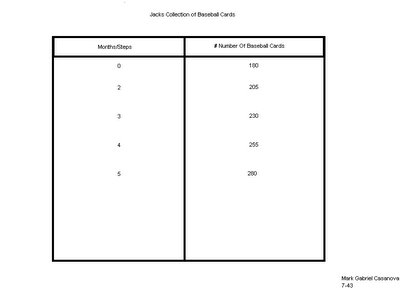## Tuesday, May 23, 2006

### THE GROWING POST!!!!!!!!!!!!!!!!!!!!!!

Mark you received 15/17 for this post

Jack collects baseball cards. Jack has 180 baseball cards in his collection. At the end of each month Jack buys 25 baseball cards to add to his collection.

a) Create a T-Chart showing how many baseball cards Jack has at the end of the next 4 months.b) Create an algebraic formula based upon the problem above
180 + 25(4) = 280

c) If Jack just turned 8 years old this month and Jack continues to buy the same amount of baseball cards each month, how many baseball cards will jack have when he turns 12?

Jack is going to have 1380 baseball cards when he turns 12.

Question #2: Use the chart to figure out the questions below.

Jackie is planning on having a pizza party with some of her friends. She is trying to figure out how many pizzas she has to order. She knows that each pizza has six slices of pizza.

A)If there is four people coming to the party then she will need 2 pizzas all together. Which count as 12 slices.

If the two people come and show up at the party then Jackie should order one more pizza to make three pizzas. Jackie should order 3 pizzas because look at the chart, and you will find 6 people and altogether will have 18 slices. Then you will divide and get the answer of 3 pizzas in total.

Question #3: Answer the questions below based upon this informationKathy has \$20 in her savings account. Each month she adds another \$15 to her savings account. In order to calculate how much money she will have in one year Kathy has created this algebraic formula: 20 + 15n = Savings amount
a) Calculate how much money Kathy will have in one year

Kathy is going to have \$200.00 in total.
b) Suppose Kathy counted wrong and she really has \$25 in her account instead of \$20. Change the algebraic formula to reflect this miscalculation.
If she could of made a mistake of having \$25.00 then the formula would be 25+15n= savings she would then have \$205.00 dollars.

Question #4: Solve the following equations. Show all of the steps that are needed.
A) 3n + 4n + 7 = 2n + 12 3n+4n+7=2n+12 3(1) +4(1) +7=2(1) +12 3+4+7=14
N= 1
B) 8n – (4 + 9) = 11 8n-(4+9) =11 8(3)-(4+9) =11 24-13=11
N= 3
C) (8 – 3) n + 7n + 8 = 4n + 40 (8-3) n+7n+8=4n+40 5n+7n+8=4n+40 5(4) +7(4) +8=4(4) +40
20+28+8=56
N= 4